xTransmittanceEncyclopedia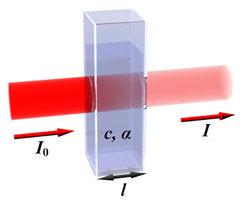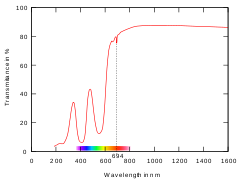In optics
Optics
Optics is the branch of physics which involves the behavior and properties of light, including its interactions with matter and the construction of instruments that use or detect it. Optics usually describes the behavior of visible, ultraviolet, and infrared light...

and spectroscopy
Spectroscopy
Spectroscopy is the study of the interaction between matter and radiated energy. Historically, spectroscopy originated through the study of visible light dispersed according to its wavelength, e.g., by a prism. Later the concept was expanded greatly to comprise any interaction with radiative...

, transmittance is the fraction of incident light (or other electromagnetic radiation
Electromagnetic radiation is a form of energy that exhibits wave-like behavior as it travels through space...

) at a specified wavelength
Wavelength
In physics, the wavelength of a sinusoidal wave is the spatial period of the wave—the distance over which the wave's shape repeats.It is usually determined by considering the distance between consecutive corresponding points of the same phase, such as crests, troughs, or zero crossings, and is a...

that passes through a sample. A related term is absorptance, or absorption factor, which is the fraction of radiation absorbed by a sample at a specified wavelength. Occasionally one also hears the terms visible transmittance (VT) and visible absorptance (VA), which are the respective fractions for the spectrum of light visible radiation. In equation form,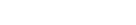where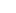is the intensity
Intensity (physics)
In physics, intensity is a measure of the energy flux, averaged over the period of the wave. The word "intensity" here is not synonymous with "strength", "amplitude", or "level", as it sometimes is in colloquial speech...

of the incident radiation and I is the intensity of the radiation coming out of the sample and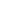and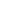are transmittance and absorptance respectively. In these equations, scattering and reflection are considered to be close to zero or otherwise accounted for. The transmittance of a sample is sometimes given as a percentage.

For liquids, transmittance is related to absorbance A (not to be confused with absorptance) as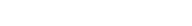In the case of gases it is customary to use natural logarithms instead, making absorbance A for gases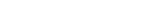From the above equation and the Beer-Lambert law
Beer-Lambert law
In optics, the Beer–Lambert law, also known as Beer's law or the Lambert–Beer law or the Beer–Lambert–Bouguer law relates the absorption of light to the properties of the material through which the light is travelling.-Equations:The law states that there is a logarithmic dependence between the...

, the transmittance for gases is thus given by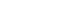,

whereis the attenuation coefficient
Attenuation coefficient
The attenuation coefficient is a quantity that characterizes how easily a material or medium can be penetrated by a beam of light, sound, particles, or other energy or matter. A large attenuation coefficient means that the beam is quickly "attenuated" as it passes through the medium, and a small...

andis the path length. For liquids e is replaced by 10.

Note that the term "transmission
Transmission coefficient
The transmission coefficient is used in physics and electrical engineering when wave propagation in a medium containing discontinuities is considered...

" refers to the physical process of radiation passing through a sample, whereas transmittance refers to the mathematical quantity.